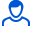Content: teorver_5592.doc (28.50 KB)

Positive responses: 0
Negative responses: 0

Refunds: 05592. x̅=10.2, n=16, σ=4. Based on the sample size n=16, the arithmetic mean x̅v=10.2 was found. Assuming that X is a normally distributed random variable, find the confidence interval that covers the unknown mathematical expectation a with reliability γ=0.99 if the general standard deviation σ=4
Detailed solution. Decorated in Microsoft Word 2003 (Quest decided to use the formula editor)
No feedback yet